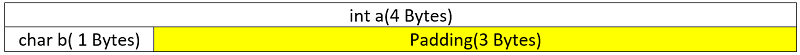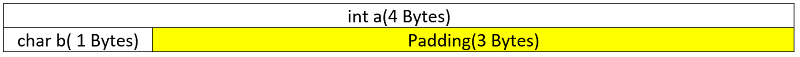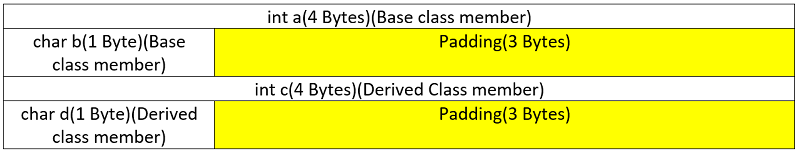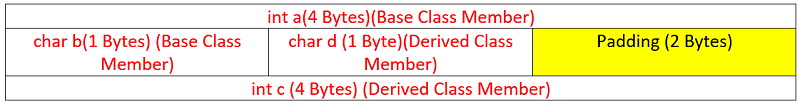# Size of a class in C++ | Padding, alignment in class | Size of derived class

In this article, we are going to see what is the size of a class and an object in C++? We will also learn what is padding, alignment provided by the compiler while defining memory for a class. Also, we have extended the idea in case of finding derived class object size as well.
Submitted by Radib Kar, on July 19, 2020

Prerequisite:

We know that a struct size is not only the summation of all the data members, rather it's the minimum sum guaranteed. The compiler adds some padding for data member alignment.

In the C++ class, things are exactly the same as a struct. But there are a few more things.

Firstly, while in C++ there are member functions, static data members. Do those have any contribution to the size of the class, objects?

The answer is no. Only the non-static data members contribute to the size of class and objects. This is because static members have only one instance which is shared among all objects. And normal member functions are like executable code which does not have size like data members.

Like in the following class,

```class A {
private:
static int i;
int a;
char b;

public:
A()
{
a = 0;
b = '#';
}
A(int aa, char bb)
{
a = aa;
b = bb;
}
int get_int()
{
cout << a << endl;
return a;
}
char get_char()
{
cout << b << endl;
return b;
}
};
```

Size of the class should be sum of all the non-static data member+ padding, which is like below:Above is the alignment of class A and that's why the size of the class is 8 Bytes. Static data members and member functions have no contribution.

Now the question is how compiler adds padding and align? The method is compiler dependent and kind of greedy. It aligns till the boundary of maximum memory allocated.

Here we find that max memory allocated is 8 Bytes, thus all the data members acquire 8 Bytes and the total size is 32 Bytes. Now the question is will it happen every time similarly?

Is it like the number of data members * max datatype size?

The answer is no. It will try to align optimally keeping the same order. To check an example please follow the article on structure size in C. Also, later in this article, we have instances of such.

## Size of a derived class

What is the size of a derived class? Of course, a derived class has all data members of the base class it inherits and does it has its own copied of those data members too. Thus size should be the size of base class data members + size of derived class data members.

Let's check the below code and the output.

In the above structure, we find that the size is 24 Bytes though the same data members have been used. This is due to the change in the order of the member declaration. In this case, the alignment and padding would be like below:

```#include <bits/stdc++.h>
using namespace std;

class Base {
protected:
static int i;
int a;
char b;

public:
Base()
{
a = 0;
b = '#';
}
Base(int aa, char bb)
{
a = aa;
b = bb;
}
int get_int()
{
cout << a << endl;
return a;
}
char get_char()
{
cout << b << endl;
return b;
}
};

class Derived : public Base {

private:
int c;
char d;

public:
Derived()
{
c = 0;
d = '#';
}
Derived(int cc, char dd)
{
c = cc;
d = dd;
}
int get_int()
{
cout << c << endl;
return c;
}
char get_char()
{
cout << d << endl;
return d;
}
};

int main()
{
Base b;
Derived d;
printf("Size of class Base: %lu\n", sizeof(Base));
printf("Size of object b: %lu\n", sizeof(b));
printf("Size of class Derived: %lu\n", sizeof(Derived));
printf("Size of object d: %lu\n", sizeof(d));
return 0;
}
```

Output:

```Size of class Base: 8
Size of object b: 8
Size of class Derived: 16
Size of object d: 16
```

So here the size of the base class object is 8 bytes, whereas the size of the derived class object is 16 Bytes.

For the base class it's similar like below:While for the derived class it's like:So the thing is the order of data members are being maintained. And since we know that the base class constructor is invoked first, that's why the base class members come first.

Now let's change the order of data member in the derived class and check the size of the class & object.

```#include <bits/stdc++.h>
using namespace std;

class Base {
protected:
static int i;
int a;
char b;

public:
Base()
{
a = 0;
b = '#';
}
Base(int aa, char bb)
{
a = aa;
b = bb;
}
int get_int()
{
cout << a << endl;
return a;
}
char get_char()
{
cout << b << endl;
return b;
}
};

class Derived : public Base {

private:
char d;
int c;

public:
Derived()
{
c = 0;
d = '#';
}
Derived(int cc, char dd)
{
c = cc;
d = dd;
}
int get_int()
{
cout << c << endl;
return c;
}
char get_char()
{
cout << d << endl;
return d;
}
};

int main()
{
Base b;
Derived d;
printf("Size of class Base: %lu\n", sizeof(Base));
printf("Size of object b: %lu\n", sizeof(b));
printf("Size of class Derived: %lu\n", sizeof(Derived));
printf("Size of object d: %lu\n", sizeof(d));
return 0;
}
```

Output:

```Size of class Base: 8
Size of object b: 8
Size of class Derived: 12
Size of object d: 12
```

Just changing the order of member we found that derived class is having size 12 Bytes now. So there must be some better alignment now.

So, as we can think of compiler went for greedy alignment and now it's able to align optimally (Remember compiler can’t change the order of data member).Above is the alignment for the Derived class and now the size is 12 Bytes, instead of 16 because of the above alignment. We saw that compiler keeps aligning greedily & that's why it aligned char b of base class member & char d, which is its member, in the same row. When it tried to align int c, it could not as only 2 bytes were left. But instead of int, if it was char only then it would have aligned in the same line.

## The virtual keyword and its effect on size

We know in the derived class it can inherit the base class as virtual too. What would be the size of the derived class in that case? Will there be any changes?

The answer is yes. There will be an additional 8 bytes which is nothing but the size of VTPR (Virtual Table pointer)

So, for the first derived class example, we got 16 Bytes, but here we have added the virtual keyword and got size 24 Bytes which is due to the size of VTPR.

```#include <bits/stdc++.h>
using namespace std;

class Base {
protected:
static int i;
int a;
char b;

public:
Base()
{
a = 0;
b = '#';
}
Base(int aa, char bb)
{
a = aa;
b = bb;
}
int get_int()
{
cout << a << endl;
return a;
}
char get_char()
{
cout << b << endl;
return b;
}
};

class Derived : virtual public Base {

private:
char d;
int c;

public:
Derived()
{
c = 0;
d = '#';
}
Derived(int cc, char dd)
{
c = cc;
d = dd;
}
int get_int()
{
cout << c << endl;
return c;
}
char get_char()
{
cout << d << endl;
return d;
}
};

int main()
{
Base b;
Derived d;
printf("Size of class Base: %lu\n", sizeof(Base));
printf("Size of object b: %lu\n", sizeof(b));
printf("Size of class Derived: %lu\n", sizeof(Derived));
printf("Size of object d: %lu\n", sizeof(d));
return 0;
}
```

Output:

```Size of class Base: 8
Size of object b: 8
Size of class Derived: 24
Size of object d: 24
```

So, here we got the idea of a normal class and object size. We learnt how the compiler aligns the data members and add padding. Also, we extended the discussion to the size of the derived class.

To end the discussion let me just throw a brain teaser. What do you think would be the size of an empty class?

Do you think it's 0?

```#include <bits/stdc++.h>
using namespace std;

class A {
};

int main()
{
printf("Size of class A: %lu\n", sizeof(A));
return 0;
}
```

Output:

```Size of class A: 1
```

Well, the size of the empty class is not 0. This is actually to ensure that two different objects will have different addresses.